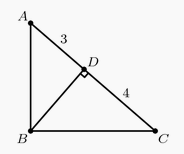#### Theorems

###### back to index

Let $R$ be the set of all possible remainders when a number of the form $2^n$, where $n$ is a non-negative integer, is divided by $1000$. Let $S$ be the sum of the elements in $R$. Find the remainder when $S$ is divided by $1000$.

In $\triangle BAC$, $\angle BAC=40^\circ$, $AB=10$, and $AC=6$. Points $D$ and $E$ lie on $\overline{AB}$ and $\overline{AC}$ respectively. What is the minimum possible value of $BE+DE+CD$?

Let $ABCDE$ be a pentagon inscribed in a circle such that $AB = CD = 3$, $BC = DE = 10$, and $AE= 14$. The sum of the lengths of all diagonals of $ABCDE$ is equal to $\frac{m}{n}$, where $m$ and $n$ are relatively prime positive integers. What is $m+n$ ?

Let $a + ar_1 + ar_1^2 + ar_1^3 + \cdots$ and $a + ar_2 + ar_2^2 + ar_2^3 + \cdots$ be two different infinite geometric series of positive numbers with the same first term. The sum of the first series is $r_1$, and the sum of the second series is $r_2$. What is $r_1 + r_2$?

The zeroes of the function $f(x)=x^2-ax+2a$ are integers .What is the sum of the possible values of a?

If integer $a$, $b$, $c$, and $d$ satisfy $ad-bc=1$. Prove $a+b$ and $c+d$ are relatively prime.

Prove for any positive integer $n$, the fraction $\frac{21n+4}{14n+3}$ cannot be further simplified.

Prove: there exists a rational number $\frac{c}{d}$, where $d<1000$, such that $$\Big[k\cdot\frac{c}{d}\Big]=\Big[k\cdot\frac{73}{100}\Big]$$ holds for every positive integer $k$ that is less than 1000. Here $\Big[x\Big]$ denotes the largest integer that is not exceeding $x$.

Prove $(n^2 -1)$ and $n$ are relatively prime.

The polynomial $x^3-ax^2+bx-2010$ has three positive integer roots. What is the smallest possible value of $a$?

Triangle $ABC$ has a right angle at $B$. Point $D$ is the foot of the altitude from $B$, $AD=3$, and $DC=4$. What is the area of $\triangle ABC$?A flagpole is originally $5$ meters tall. A hurricane snaps the flagpole at a point $x$ meters above the ground so that the upper part, still attached to the stump, touches the ground $1$ meter away from the base. What is $x$?

A right triangle has perimeter $32$ and area $20$. What is the length of its hypotenuse?

A quadratic equation $ax^2 - 2ax + b = 0$ has two real solutions. What is the average of these two solutions?

A triangle with side lengths in the ratio $3 : 4 : 5$ is inscribed in a circle with radius 3. What is the area of the triangle?

Let $a$ and $b$ be the roots of the equation $x^2-mx+2=0$. Suppose that $a+\frac1b$ and $b+\frac1a$ are the roots of the equation $x^2-px+q=0$. What is $q$?

The quadratic equation $x^2+mx+n=0$ has roots twice those of $x^2+px+m=0$, and none of $m,n,$ and $p$ is zero. What is the value of $\frac{n}{p}$?

Nicky is studying biology and has a tank of $17$ lizards. In one day, he can either remove $5$ lizards or add $2$ lizards to his tank. What is the minimum number of days necessary for Nicky to get rid of all of the lizards from his tank?

Let polynomials $P(x)$, $Q(x)$, $R(x)$, and $S(x)$ satisfy: $$P(x^5) + xQ(x^5)+x^2R(x^5)=(x^4+x^3+x^2+x+1)S(x)$$ Prove: $(x-1) | P(x)$

Let $\alpha$ and $\beta$ be two real roots of the equation $x^2 + x - 4=0$. Find the value of $\alpha^2 - 5\beta + 10$ without computing the value of $\alpha$ and $\beta$.

Solve the following equations for all real numbers $r, s, t$: $$\begin{array}{rl} rst &=30\\ rs+st+tr &=-11\\ r+s+t &=-4 \end{array}$$

Let $\gamma_i$ and $\overline{\gamma_i}$ be the 10 zeros of $x^{10}+(13x-1)^{10}$, where $i=1, 2, 3, 4, 5$. Compute $$\frac{1}{\gamma_1 \overline{\gamma_1}}+\frac{1}{\gamma_2 \overline{\gamma_2}}+\cdots+\frac{1}{\gamma_5 \overline{\gamma_5}}$$

What is the sum of all of the roots of $(2x + 3) (x - 4) + (2x + 3) (x - 6) = 0$?

Given a triangle with side lengths 15, 20, and 25, find the triangle's smallest height.

The inradius of triangle $ABC$ is $1$ and the side lengths of $ABC$ are all integers. Prove that triangle $ABC$ is right-angled.